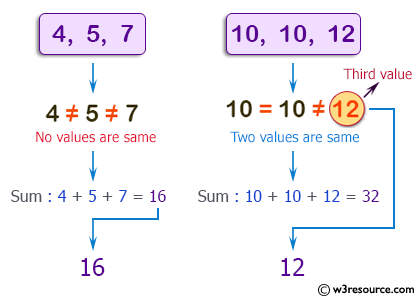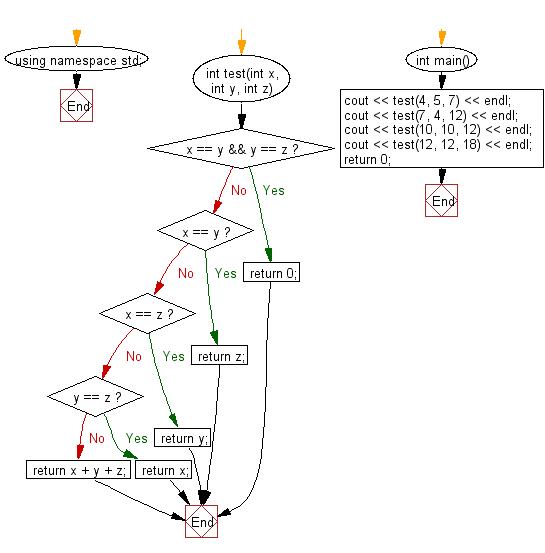﻿ C++ : If the two values are the same, return the third value# C++ Exercises: Compute the sum of three given integers. If the two values are same return the third value

## C++ Basic Algorithm: Exercise-52 with Solution

Write a C++ program to compute the sum of three given integers. If the two values are same return the third value.

Sample Solution:

C++ Code :

``````#include <iostream>
using namespace std;

int test(int x, int y, int z)
{
if (x == y && y == z) return 0;
if (x == y) return z;
if (x == z) return y;
if (y == z) return x;
return x + y + z;
}

int main()
{
cout << test(4, 5, 7) << endl;
cout << test(7, 4, 12) << endl;
cout << test(10, 10, 12) << endl;
cout << test(12, 12, 18) << endl;
return 0;
}
``````

Sample Output:

```16
23
12
18
```

Pictorial Presentation:Flowchart:C++ Code Editor:

Contribute your code and comments through Disqus.

What is the difficulty level of this exercise?

﻿